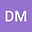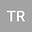A General Mathematical Approach On the Selection of Auxiliary Linear Operator and Initial Guess for Homotopy Methods
••Dilip Kumar Maiti
Vidyasagar University

Corresponding Author:d_iitkgp@yahoo.com

Author Profile## Abstract

In this study we propose a general mathematical algorithm for the selection of aux- iliary linear operator () and initial guess 0(), which are the principal parts of Homotopy methods: Homotopy Perturbation Method (HPM) and Homotopy Anal- ysis Method (HAM). We assume the coefficients of derivatives involved in () as a functions of auxiliary roots of () = 0. Based on the residual error minimization we compute unknown roots and thereby obtain the best fitted optimal linear operator. Additionally, from the efficiency standpoint, we suggest discretize the exact square residual using the Simson’s 31 algorithm. We applied our algorithms to six nonlin- ear problems: (i) two nonlinear initial value problem (IVP) (ii) two highly nonlinear BVPs with quadratic and cubic nonlinearity, (iii) Bessel equation of zero-order and (iv) A singular and highly nonlinear BVP (for fluid electrohydrodynamics). We then compare our technique’s accuracy and efficiency to other existing analytical and nu- merical methods. It demonstrates that our best fitted optimal linear operator is much more efficient, important (than the artificial controlling parameters or functions of optimal HAM) and self-sufficient for the convergence of series solutions over the whole domain, specially for IVP. Also, an effort is made to search the best () for different choices of real roots and by means of fastest converges of the solution. Our approach is more effective, straightforward and easy to use when applied to many nonlinear problems arises in science and engineering, and using our propose ap- proach homotopy methods (HAM and HPM) will be more powerful.